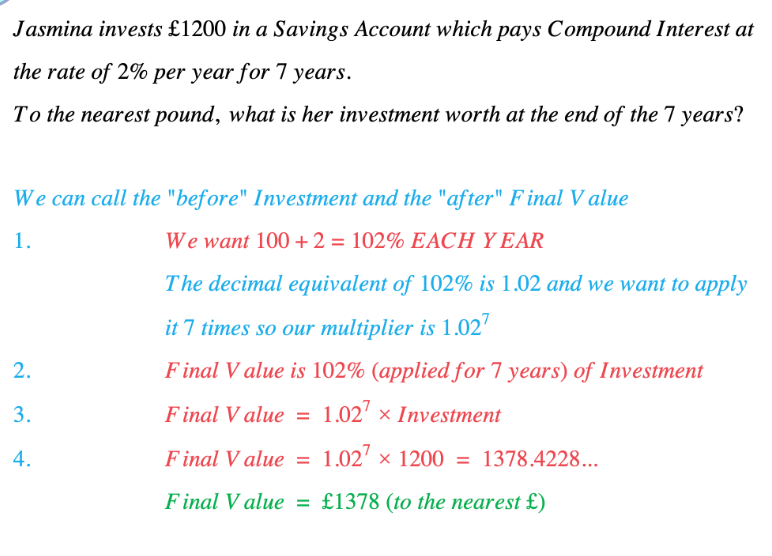# Edexcel IGCSE Maths复习笔记 1.9.1 Compound Interest

Edexcel IGCSE Maths复习笔记 1.9.1 Compound Interest

#### What is compound interest?

• Compound interest is where interest is paid on the interest from the year (or whatever time frame is being used) before as well as on the original amount
• This is different from simple interest where interest is only paid on the original amount

#### How do you work with compound interest?

• For COMPOUND changes (can be a decrease as well as an increase):
• Keep multiplying by the decimal equivalent of the percentage you want

• Otherwise do the same as normal:
• Identify “before” & “after” quantities
• FIND percentage we want:
• Increase – ADD percentage to 100
• Decrease – SUBTRACT from 100

• Write down a STATEMENT connecting “before” and “after”:
• “after is a percentage of before”

• Write down the statement as an EQUATION using decimal equivalent
• remember “is” means “=”

• SUBSTITUTE and SOLVE

#### Exam Tip

This method works for any Compound Change – increase or decrease.Remembering "✕ m ✕ m ✕ m = ✕ m3" can make life a lot quicker:It is usually much easier to multiply by decimal equivalent raised to a power than to multiply by the decimal equivalent several times in a row.

Worked Example